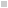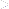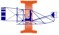####BACKHomeHistory WingAdventure WingExhibits & ProgramsCompany StoreInformation Desk###The Angle of      Incidence(You are here.)###Available in Française, Español, Português, Deutsch, Россию, 中文, 日本, and others.

In Their Own Wordsn Wilbur's time, the "angle of incidence" was what we now call the "angle of attack" -- the angle at which the wing of an airplane meets the wind. This is difficult to measure in the field with any accuracy, so Wilbur suggests that it might be measured indirectly by using three variables that can be more easily determined, then using a chart to look up the angle. Besides the subject at hand, this article reveals three things concerning Wilbur's character, his focus, and his methods. First it shows that Wilbur, although he is a newcomer to field of aeronautics, has the self-confidence to challenge other, more experienced scientists. Second, the movements he describes are the sorts that an unpowered glider would make -- Wilbur is focused on soaring flight. And finally, he paints a series of triangles and angles to make his analysis. Wilbur is using trigonometry to analyze the phenomenon of flight. It has been said that the invention of the airplane was a triumph of trigonometry, and this is the first battle fought and won.

If the term “angle of incidence," so frequently used in aeronautical discussions, could be confined to a single definite meaning, viz., the angle at which aeroplane and wind actually meet, much error and confusion would be averted. But many of the best writers use this term loosely and inexactly, with the result that- their calculations and explanations of phenomena are thereby often rendered of little value, and students are misled.

If a plane be held stationary in a horizontal wind (Fig. I), the angle of incidence A C D will be equal to the angle with the horizon. But if the wind has an upward trend, the angle of incidence will be increased to A C D2. A calculation based on the assumption that A C D still remained the true angle would, of course, be seriously in error.

If it be assumed (Fig. 2) that the wind moves from D to C, while the plane moves from D2 to C, the angle of incidence will become A C D3. The wind having an apparent horizontal speed of F C + E C, which is equal to F2C as compared with a vertical speed of D2F, the wind will appear to strike the plane from the direction D3C, thus making its angle of incidence A C D3 instead of A C D2. Taking still another case (Fig. 3), in which the wind moves from D2 to C, while the plane is rising from C to C2 the angle of incidence will be A C X. The horizontal speed of the wind will be F C. and its apparent vertical speed D2 F - C C2 = X F, so that the wind will appear to strike the plane from the direction X C. If the plane had fallen from C to C3 the angle of incidence would have been A C X2, since the vertical motion would now have been D3 F + C C3 = X2 F. A study of the foregoing will lead to the conclusion that in order to obtain the angle of incidence it is first necessary to know correctly (1) the angle of the plane with the horizon; (2) the horizontal speed of the wind; (3) the vertical speed of the wind; (4) the horizontal speed of the plane; (5) the vertical spend of the plane. Now, since the probability of obtaining five correct simultaneous measurements is very slight when the observation is taken in the open air and since the soaring angle is not. supposed to exceed three degrees, it is evident that calculations based upon observations of soaring birds are of exceedingly doubtful value.

But though direct attempts to calculate the angle of incidence promise little for accuracy, another method remains by which approximately correct results may be obtained. The tables of Langley, Lilionthal, Chanutc, and others give with a good degree of accuracy, the vertical component of the normal pressure at all angles and speeds, both for planes and curved surfaces. Now, since the elements of these tables are four, viz., area, speed, angle, and lift, it follows that three factors being known the fourth may readily be obtained. The angle of incidence may be calculated in any case where speed, lift, and area are commensurable. Of these, area may be obtained by actual measurement. Lift is equal to weight supported, and can also be exactly measured. Speed alone requires to be calculated at the instant the observation is made. Thus the opportunity for error is reduced to a single item, and this the one which affects the result least seriously in case of a slight error. It is easier to measure speeds than angles and errors are less costly. There is, of course, a possibility of error in the tables, but the results have been confirmed by the experiments of independent observers, and are believed to be substantially correct. Calculations of the angle of incidence based upon this plan would scarcely be so seriously in error as  that of a  well-known writer based upon observations of soaring gulls, in which he obtained from his measurements of angles and speeds an angle of incidence which called for an upward lift of nearly three pounds upon a two-pound bird. It was his idea that the excess of lift was expended in raising the bird above its original level. This, however, is scarcely a satisfactory explanation of how a lift of three pounds could be applied to a two-pound bird in contradiction of the law that action and reaction are always equal. It would lie more reasonable to assume that the excessive lift never existed, but that the upward motion of the bird kept the angle of incidence (Fig. 3) reduced to a point where the lift was just equal to the bird's weight, all of the time.

If students of aeronautical problems will constantly bear in mind that (1) area of surface; (2) weight or lift; (3) relative speed are the only factors concerned in determining the angle of incidence, many errors will be detected and much trouble saved. It is clear that a bird, instead of possessing flull control of its angle of incidence (as writers sometimes assume), is, in fact, almost powerless to change it. The bird is really itself controlled by a kind of automatic governor, for a gust of wind which tends to increase the lift; is balked by the Fact that the weight of the bird is not sufficient to furnish the required reaction, and the bird must either float back horizontally with a speed equal to the increase in the velocity of the wind, or it will involuntarily be raised at a speed sufficient to keep its angle of incidence at a point where the increased lifting pressure naturally due to the increased speed will be exactly counterbalanced. Nevertheless the bird can, to a limited extent, affect its area, its weight, and its speed, and thus indirectly its angle of incidence also. Its area may be reduced (and its angle increased) by partly folding its wings, but as, in actual practice, a folding of the wings always accom­panies an increase of relative velocity (which produces a decrease in angle), the real effect is to preserve the original angle instead of changing it. The apparent weight may be momentarily increased by a sudden curve in the direction of motion, in which case the momentum of its body acts to produce a centrifugal force which increases the downward pressure on its wings, and this requires an increase in the angle of incidence to furnish the required extra support. Speed may be controlled by increasing or decreasing the elevation of the bird or its rate of fall. But. none of these changes in speed, area or weight, quickly and permanently affect its angle of incidence; The bird can at will vary its angle with the horizon, even to the extent of three hundred and sixty degrees in loss than a second, as when it turns a complete somersault in the air: but its angle of incidence probably does not vary as much as one-twentieth of that amount in the same case. It is very important to distinguish carefully between the angle of incidence, and the angle with the horizon, as the latter has nothing at all per  se to do with the former. By keeping the distinction, carefully in mind, the student will quickly obtain new ideas of the correct explanation of the action of the Penaud tail used by Lilienthal, Pilcher, and Chanute; and correct the sequence of cause and effect in many explanations of phenomena which occur in aeronautical writings.

Since the formulation of a principle into a rule often serves to fix it more prominently in the mind, the writer ventures to offer the following:

Rule.—The angle of incidence is fixed by area, weight, and speed alone. It varies directly as the weight, and inversely as the area and speed, though not in exact ratio.